CBSE Class 10 Sample Paper for 2021 Boards - Maths Standard

Class 10
Solutions of Sample Papers for Class 10 Boards

## SIMILAR FIGURES The ratio of two corresponding sides in similar figures is called the scale factor.

Note: Check more Case Based Questions for - Triangles Class 10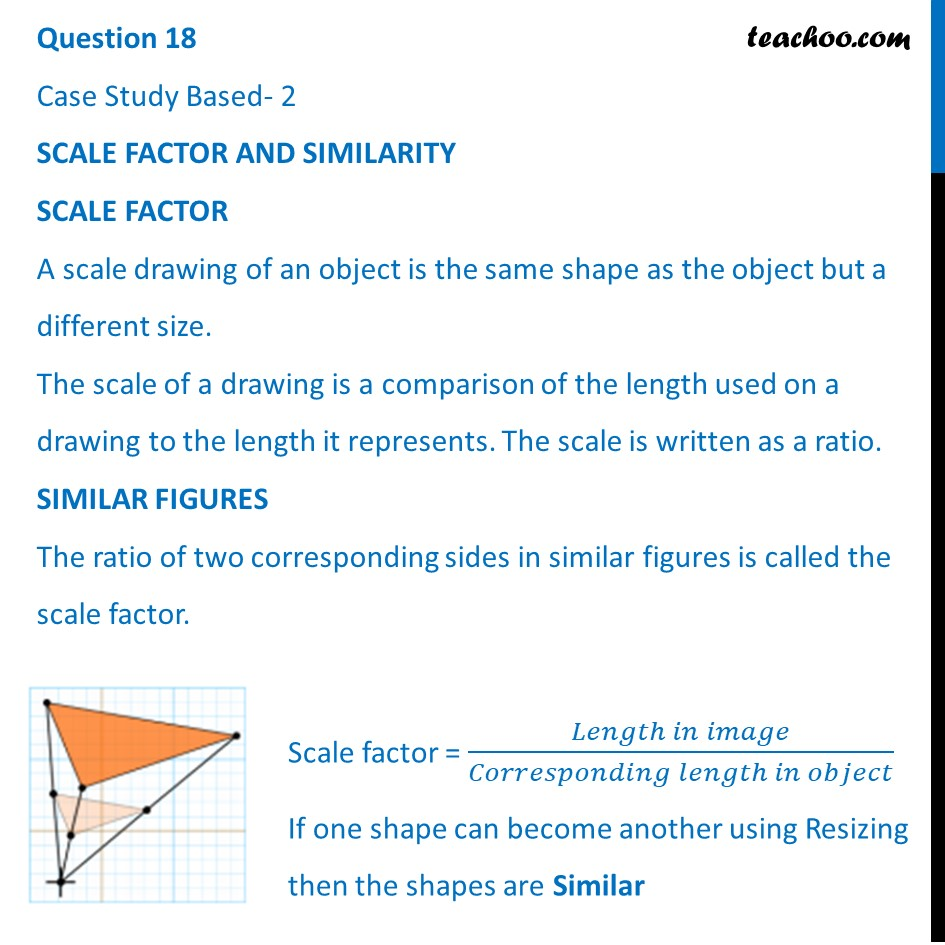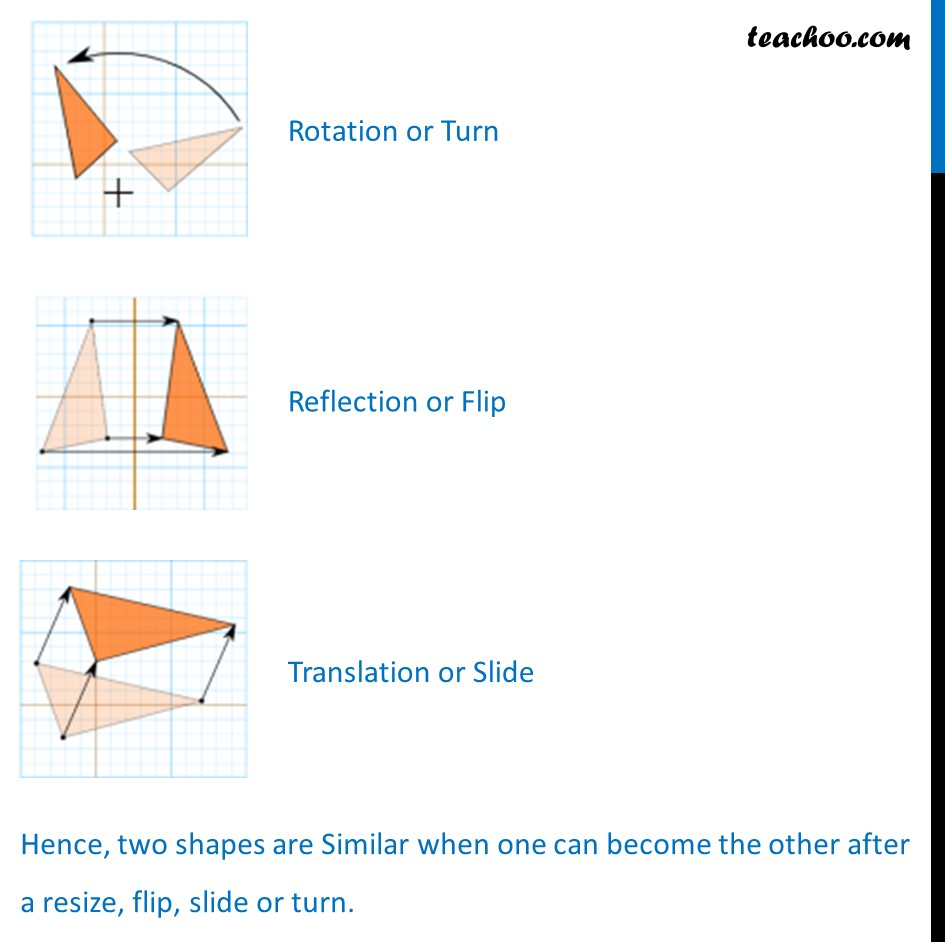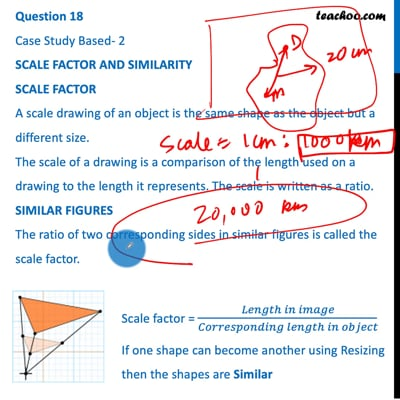This video is only available for Teachoo black users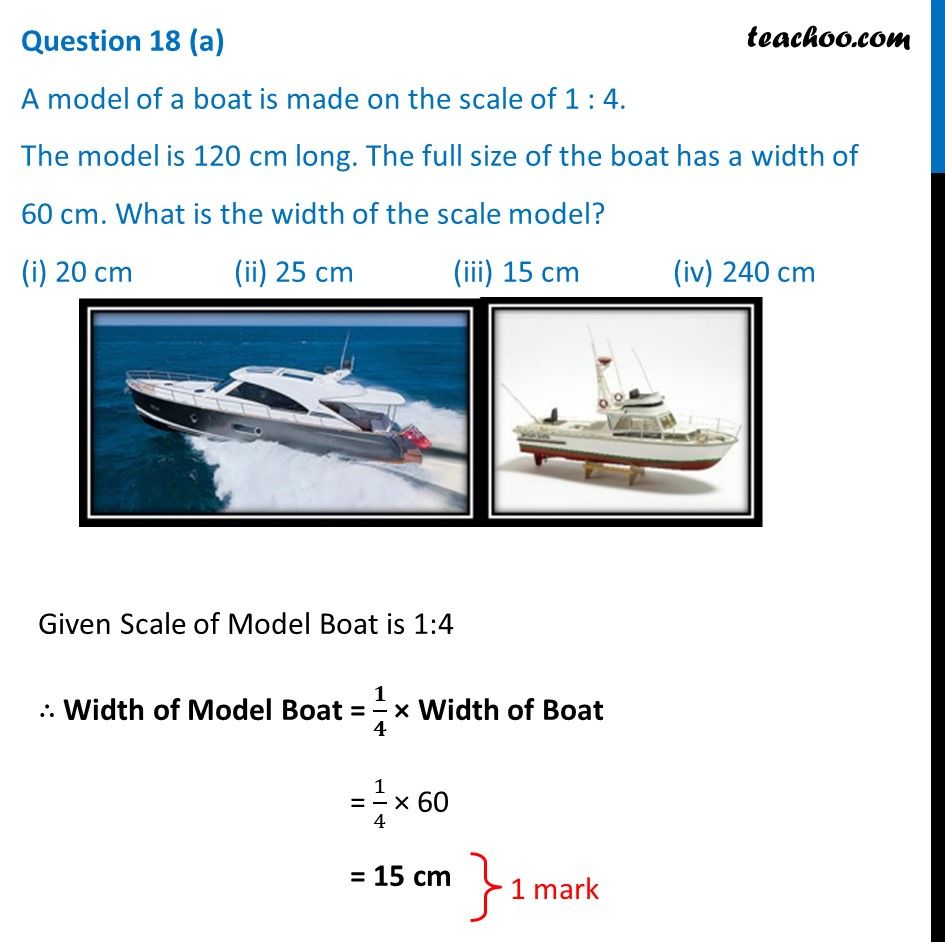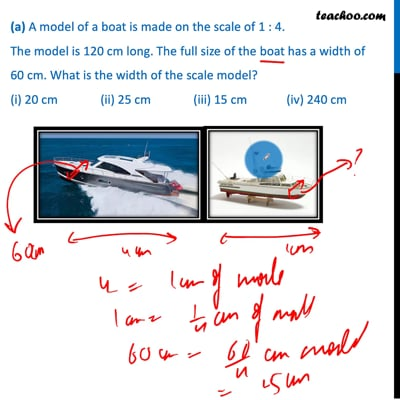This video is only available for Teachoo black users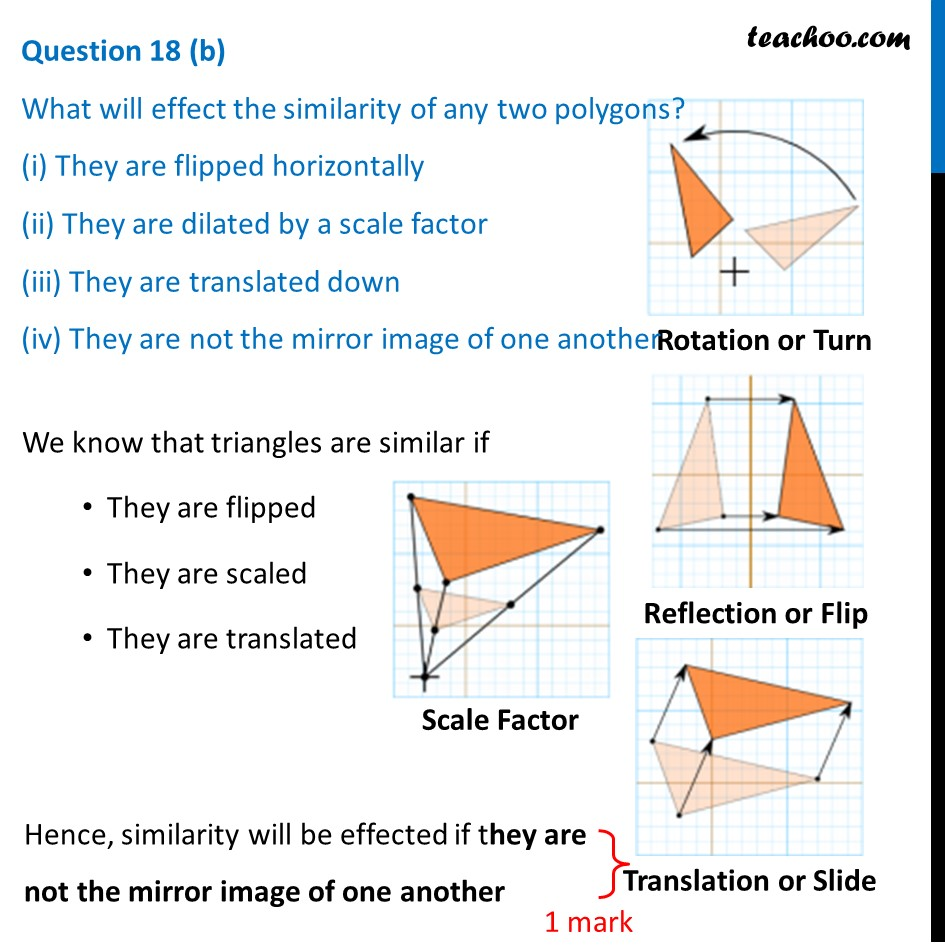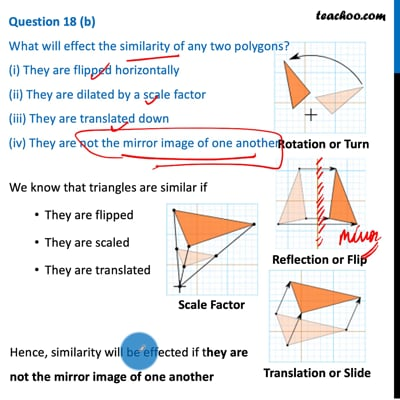This video is only available for Teachoo black users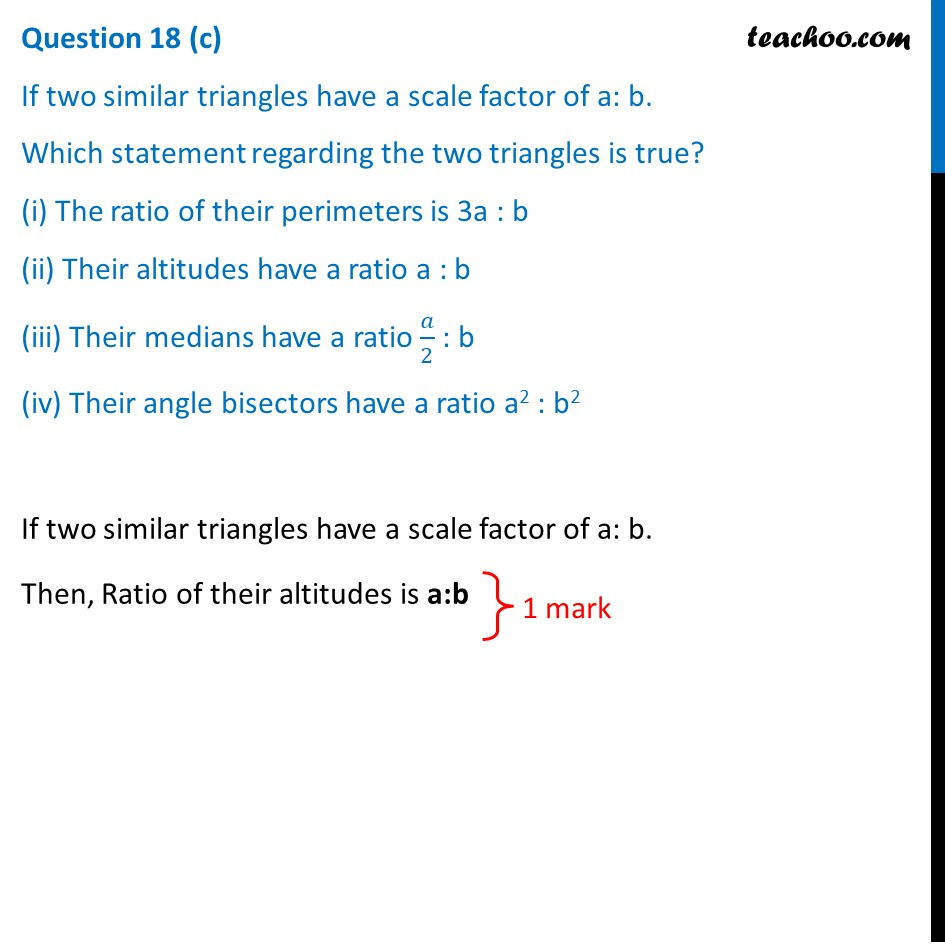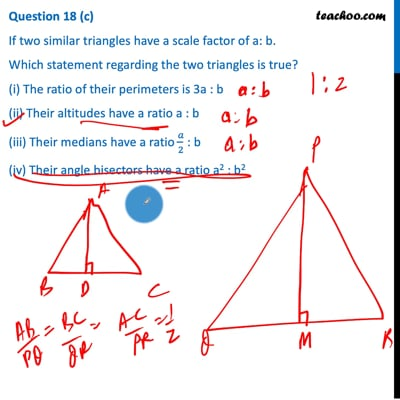This video is only available for Teachoo black users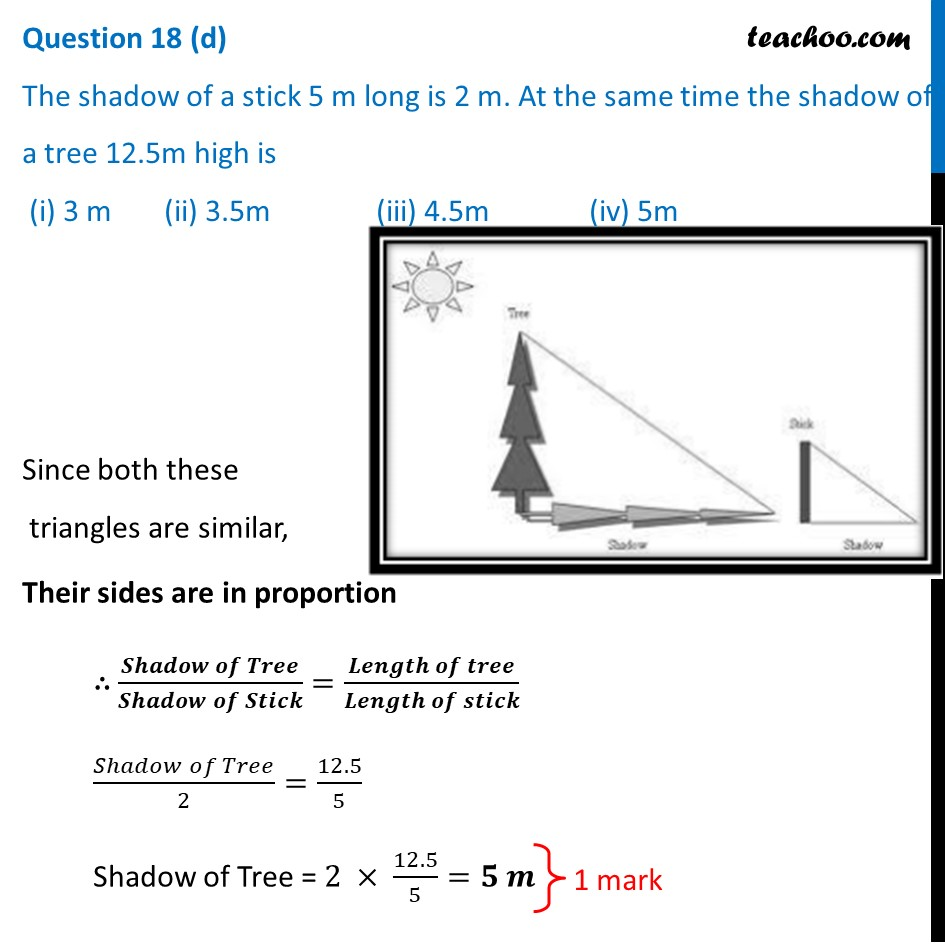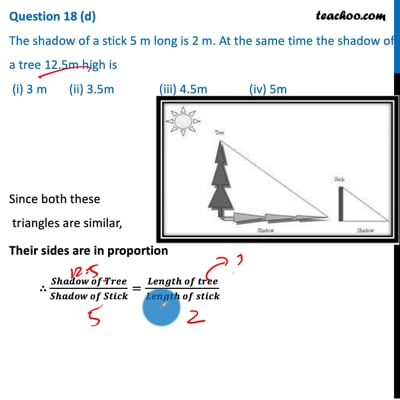This video is only available for Teachoo black users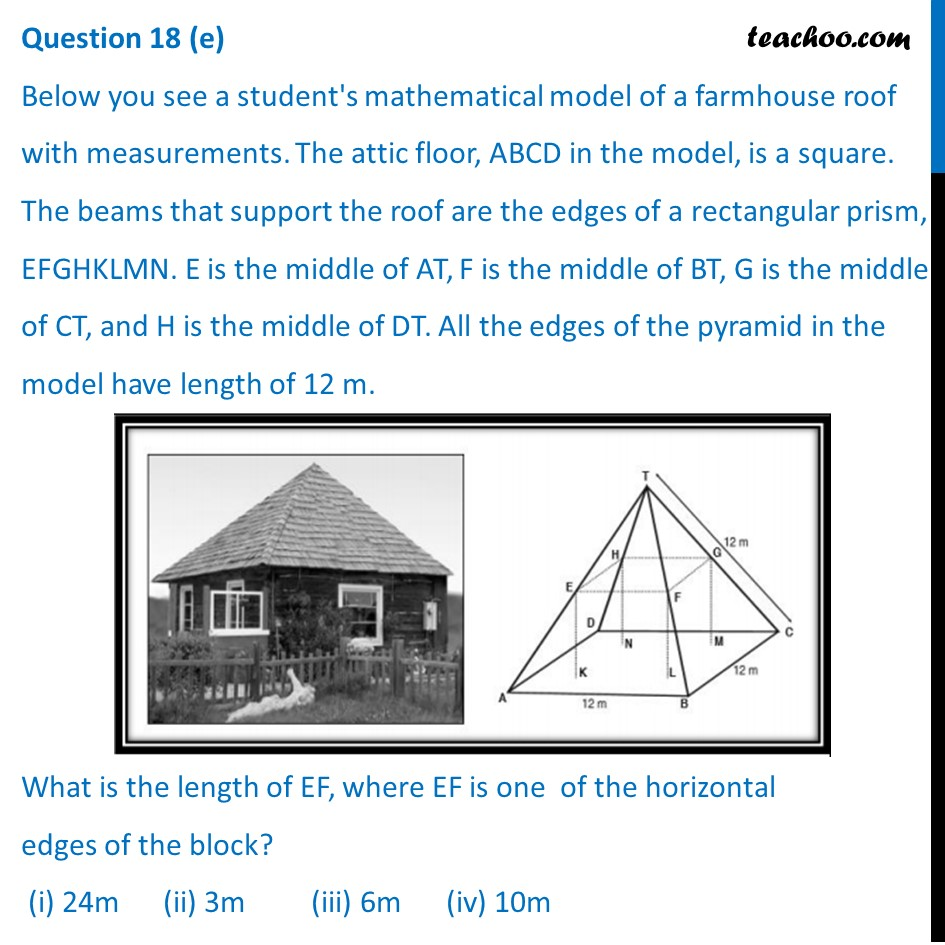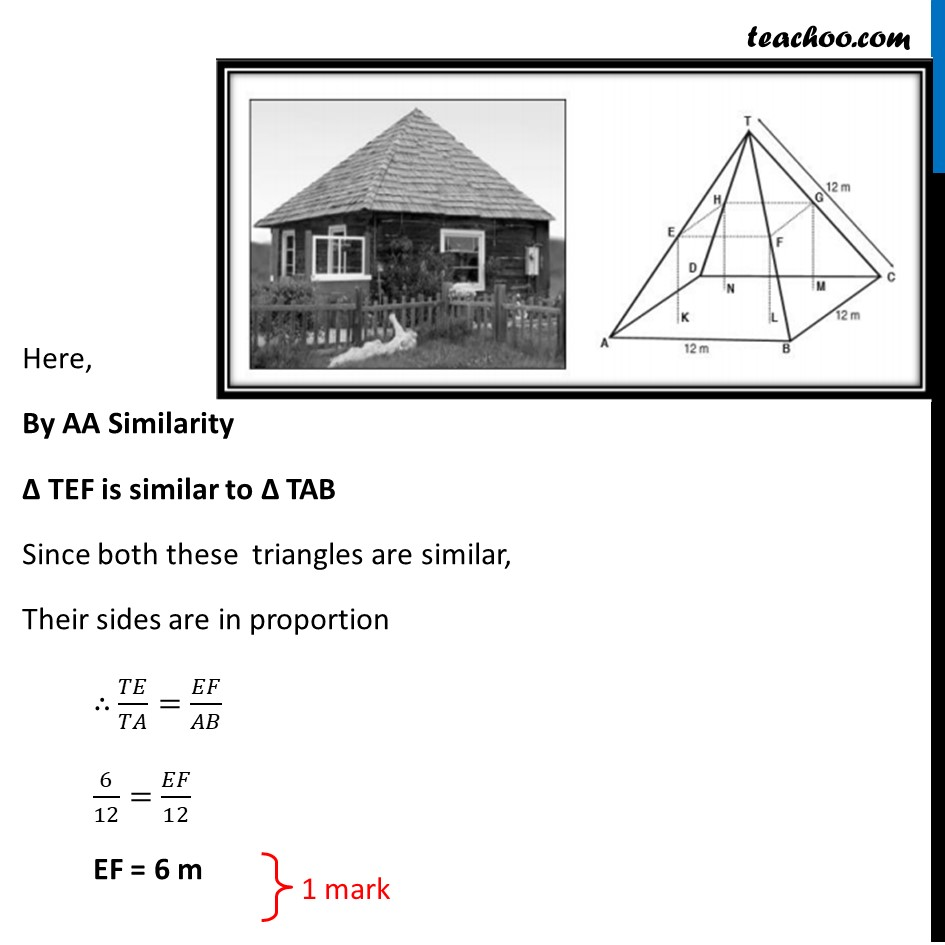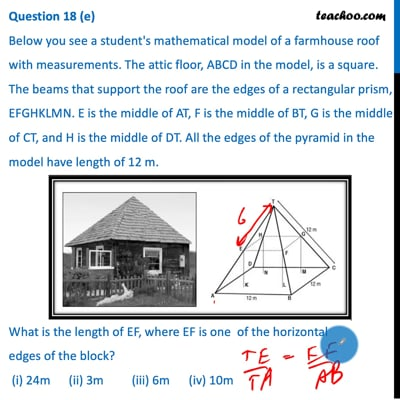This video is only available for Teachoo black users

Note: Check more Case Based Questions for - Triangles Class 10

Introducing your new favourite teacher - Teachoo Black, at only ₹83 per month

### Transcript

Question 18 Case Study Based- 2 SCALE FACTOR AND SIMILARITY SCALE FACTOR A scale drawing of an object is the same shape as the object but a different size. The scale of a drawing is a comparison of the length used on a drawing to the length it represents. The scale is written as a ratio. SIMILAR FIGURES The ratio of two corresponding sides in similar figures is called the scale factor. Scale factor = (𝐿𝑒𝑛𝑔𝑡ℎ 𝑖𝑛 𝑖𝑚𝑎𝑔𝑒)/(𝐶𝑜𝑟𝑟𝑒𝑠𝑝𝑜𝑛𝑑𝑖𝑛𝑔 𝑙𝑒𝑛𝑔𝑡ℎ 𝑖𝑛 𝑜𝑏𝑗𝑒𝑐𝑡) If one shape can become another using Resizing then the shapes are Similar Rotation or Turn Reflection or Flip Translation or Slide Hence, two shapes are Similar when one can become the other after a resize, flip, slide or turn. (a) A model of a boat is made on the scale of 1 : 4. The model is 120 cm long. The full size of the boat has a width of 60 cm. What is the width of the scale model? (i) 20 cm (ii) 25 cm (iii) 15 cm (iv) 240 cm Given Scale of Model Boat is 1:4 ∴ Width of Model Boat = 𝟏/𝟒 × Width of Boat = 1/4 × 60 = 15 cm (b) What will effect the similarity of any two polygons? (i) They are flipped horizontally (ii) They are dilated by a scale factor (iii) They are translated down (iv) They are not the mirror image of one another We know that triangles are similar if They are flipped They are scaled They are translated Hence, similarity will be effected if they are not the mirror image of one another Hence, similarity will be effected if they are not the mirror image of one another (c) If two similar triangles have a scale factor of a: b. Which statement regarding the two triangles is true? (i) The ratio of their perimeters is 3a : b (ii) Their altitudes have a ratio a : b (iii) Their medians have a ratio 𝑎/2 : b (iv) Their angle bisectors have a ratio a2 : b2If two similar triangles have a scale factor of a: b. Then, Ratio of their altitudes is a:b (d) The shadow of a stick 5 m long is 2 m. At the same time the shadow of a tree 12.5m high is (i) 3 m (ii) 3.5m (iii) 4.5m (iv) 5m Since both these triangles are similar, Their sides are in proportion ∴ (𝑆ℎ𝑎𝑑𝑜𝑤 𝑜𝑓 𝑇𝑟𝑒𝑒)/(𝑆ℎ𝑎𝑑𝑜𝑤 𝑜𝑓 𝑆𝑡𝑖𝑐𝑘)=(𝐿𝑒𝑛𝑔𝑡ℎ 𝑜𝑓 𝑡𝑟𝑒𝑒)/(𝐿𝑒𝑛𝑔𝑡ℎ 𝑜𝑓 𝑠𝑡𝑖𝑐𝑘) (𝑆ℎ𝑎𝑑𝑜𝑤 𝑜𝑓 𝑇𝑟𝑒𝑒)/2=12.5/5 Shadow of Tree = 2 × 12.5/5=𝟓 𝒎 (e) Below you see a student's mathematical model of a farmhouse roof with measurements. The attic floor, ABCD in the model, is a square. The beams that support the roof are the edges of a rectangular prism, EFGHKLMN. E is the middle of AT, F is the middle of BT, G is the middle of CT, and H is the middle of DT. All the edges of the pyramid in the model have length of 12 m. What is the length of EF, where EF is one of the horizontal edges of the block? (i) 24m (ii) 3m (iii) 6m (iv) 10mHere, Δ TEF is similar to Δ TAB Since both these triangles are similar, Their sides are in proportion ∴ 𝑇𝐸/𝑇𝐴=𝐸𝐹/𝐴𝐵 6/12=𝐸𝐹/12 EF = 6 m